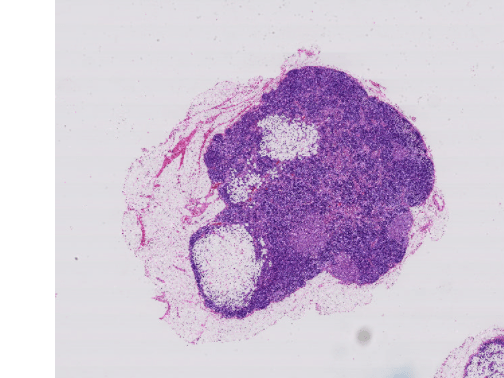# Process Blocked Images Efficiently Using Partial Images or Lower Resolutions

This example shows how to process a blocked image quickly using two strategies that enable computations on smaller representative samples of the high-resolution image.

Processing blocked images can be time consuming, which makes iterative development of algorithms prohibitively expensive. There are two common ways to shorten the feedback cycle: iterate on a lower resolution image or iterate on a partial region of the blocked image. This example demonstrates both of these approaches for creating a segmentation mask for a blocked image.

If you have Parallel Computing Toolbox™ installed, then you can further accelerate the processing by using multiple workers.

Create a `blockedImage` object using a modified version of image "tumor_091.tif" from the CAMELYON16 data set. The original image is a training image of a lymph node containing tumor tissue. The original image has eight resolution levels, and the finest level has resolution 53760-by-61440. The modified image has only three coarse resolution levels. The spatial referencing of the modified image has been adjusted to enforce a consistent aspect ratio and to register features at each level.

`bim = blockedImage("tumor_091R.tif");`

Display the blocked image by using the `bigimageshow` function.

`bigimageshow(bim);`### Accelerate Processing Using Lower Resolution Image

Many blocked images contain multiple resolution levels, including coarse lower resolution versions of the finest high-resolution image. In general, the distribution of individual pixel values should be roughly equal across all the levels. Leveraging this assumption, you can compute global statistics at a coarse level and then use the statistics to process the finer levels.

Extract the image at the coarsest level, then convert the image to grayscale.

```imLowRes = gather(bim); imLowResGray = im2gray(imLowRes);```

Threshold the image into two classes and display the result.

```thresh = graythresh(imLowResGray); imLowResQuant = imbinarize(imLowResGray,thresh); imshow(imLowResQuant)```Validate on the largest image. Negate the result to obtain a mask for the stained region.

`bq = apply(bim,@(bs)~imbinarize(rgb2gray(bs.Data),thresh));`

Visualize the result at the finest level.

`bigimageshow(bq,CDataMapping="scaled");`### Accelerate Processing Using Partial Regions of Blocked Image

Another approach while working with large images is to extract a smaller region with features of interest. You can compute statistics from the ROI and then use the statistics to process the entire high-resolution image.

Zoom in on a region of interest.

```bigimageshow(bim); xlim([2400,3300]) ylim([900 1700])```Extract the region being shown from the finest level.

```xrange = xlim; yrange = ylim; imRegion = getRegion(bim,[900 2400],[1700 3300],Level=1); imshow(imRegion);```Prototype with this region then display the results.

```imRegionGray = rgb2gray(imRegion); thresh = graythresh(imRegionGray); imLowResQuant = ~imbinarize(imRegionGray,thresh); imshow(imLowResQuant)```Validate on the full blocked image and display the results.

```bq = apply(bim,@(bs)~imbinarize(rgb2gray(bs.Data),thresh)); bigimageshow(bq,CDataMapping="scaled");```### Accelerate Processing Using Parallel Computing Toolbox

If you have the Parallel Computing Toolbox installed, then you can distribute the processing across multiple workers to accelerate the processing. To try processing the image in parallel, set the `runInParallel` variable to `true`.

```runInParallel = false; if runInParallel % Location for output, which should be accessible on the client and all % workers and should be empty outDir = tempname; % Open a pool p = gcp; % Ensure workers are on the same folder as the file to be able to % access it using just the relative path sourceDir = fileparts(which("tumor_091R.tif")); spmd cd(sourceDir) end % Run in parallel bq = apply(bim, ... @(bs)~imbinarize(rgb2gray(bs.Data),thresh),UseParallel=true, ... OutputLocation=outDir); end```